# Problem: At the instant the displacement of a 2.00 kg object relative to the origin is    = (2.00 m)  + (4.00 m)  -(3.00 m) , its velocity is  = -(6.00 m/s)  + (3.00 m/s)  + (3.00 m/s)  and it is subject to a force = (6.00 N)  -(8.00 N)  + (4.00 N) . Find (a) the acceleration of the object, (b) the angular momentum of the object about the origin,(c) the torque about the origin acting on the object, and (d) the angle between the velocity of the object and the force acting on the object.

###### FREE Expert Solution

In this problem, we have both cross and dot products of vectors.

(a)

Newton's second law:

$\overline{){\mathbf{\Sigma }}{\mathbf{F}}{\mathbf{=}}{\mathbf{m}}{\mathbf{a}}}$

m = 2.00kg

F = = (6.00 N)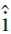$-$(8.00 N)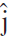$+$ (4.00 N)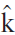We are dividing a vector by a scalar.

79% (104 ratings)###### Problem Details

At the instant the displacement of a 2.00 kg object relative to the origin is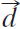= (2.00 m)$+$ (4.00 m)$-$(3.00 m), its velocity is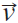= $-$(6.00 m/s)$+$ (3.00 m/s)$+$ (3.00 m/s)and it is subject to a force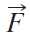= (6.00 N)$-$(8.00 N)$+$ (4.00 N). Find

(a) the acceleration of the object,

(b) the angular momentum of the object about the origin,

(c) the torque about the origin acting on the object, and

(d) the angle between the velocity of the object and the force acting on the object.